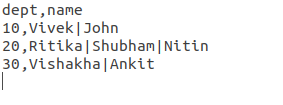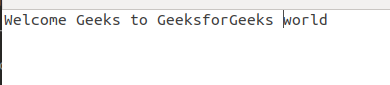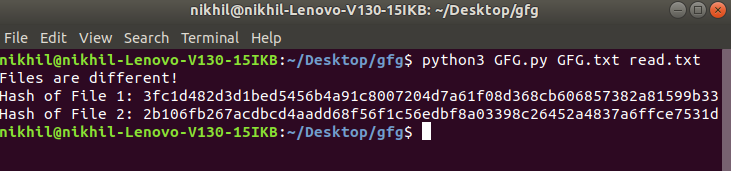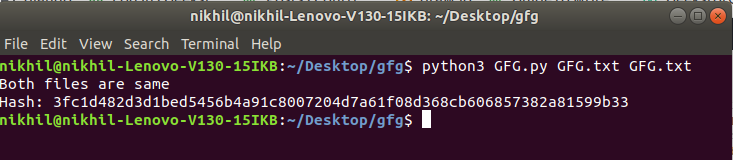# Compare two files using Hasing in Python

In this article, we would be creating a program that would determine, whether two files provided to it are the same or not. By the same means that their contents are the same or not (excluding any metadata). We would be using Cryptographic Hashes for this purpose. A cryptographic hash function is a function that takes in input data and produces a statistically unique output, which is unique to that particular set of data. We would be using this property of Cryptographic hash functions to identify the contents of two files, and then would compare that to determine whether they are same or not.

Note: The probability of getting the same has for two different data set is very very low. And even then the good cryptographic hash functions are made so that hash collisions are accidental rather than intentional.

We would be using SHA256 (Secure hash algorithm 256) as a hash function in this program. SHA256 is very resistant to collisions. We would be using `hashlib` library’s `sha256()` to use the implementation of the function in python.

`hashlib `module is preinstalled in most python distributions. If it doesn’t exists in your environment, then you can get the module by running the following command in the command–

```pip install hashlib
```

Below is the implementation.

Text File 1:Text File 2:`import` `sys ` `import` `hashlib ` `  `  ` `  `def` `hashfile(``file``): ` `  `  `    ``# A arbitrary (but fixed) buffer  ` `    ``# size (change accordingly) ` `    ``# 65536 = 65536 bytes = 64 kilobytes  ` `    ``BUF_SIZE ``=` `65536`  `  `  `    ``# Initializing the sha256() method ` `    ``sha256 ``=` `hashlib.sha256() ` `  `  `    ``# Opening the file provided as ` `    ``# the first commandline arguement ` `    ``with ``open``(``file``, ``'rb'``) as f: ` `         `  `        ``while` `True``: ` `             `  `            ``# reading data = BUF_SIZE from ` `            ``# the file and saving it in a ` `            ``# variable ` `            ``data ``=` `f.read(BUF_SIZE) ` `  `  `            ``# True if eof = 1 ` `            ``if` `not` `data: ` `                ``break` `      `  `            ``# Passing that data to that sh256 hash ` `            ``# function (updating the function with ` `            ``# that data) ` `            ``sha256.update(data) ` `  `  `      `  `    ``# sha256.hexdigest() hashes all the input ` `    ``# data passed to the sha256() via sha256.update() ` `    ``# Acts as a finalize method, after which ` `    ``# all the input data gets hashed hexdigest() ` `    ``# hashes the data, and returns the output ` `    ``# in hexadecimal format ` `    ``return` `sha256.hexdigest() ` ` `  `# Calling hashfile() function to obtain hashes ` `# of the files, and saving the result ` `# in a variable ` `f1_hash ``=` `hashfile(sys.argv[``1``]) ` `f2_hash ``=` `hashfile(sys.argv[``2``]) ` `  `  `# Doing primitive string comparison to  ` `# check whether the two hashes match or not ` `if` `f1_hash ``=``=` `f2_hash: ` `    ``print``(``"Both files are same"``) ` `    ``print``(f``"Hash: {f1_hash}"``) ` ` `  `else``: ` `    ``print``(``"Files are different!"``) ` `    ``print``(f``"Hash of File 1: {f1_hash}"``) ` `    ``print``(f``"Hash of File 2: {f2_hash}"``) `

Output:

For Different Files as Input:For Same Files as Input:Explanation:-

We take in input the filenames (via command-line argument), therefore the file paths must be provided from the command line. The function `hashfile()` is defined, to deal with arbitrary file sizes without running out of memory. As if we pass all the data in a file to the `sha256.update()` function, it doesn’t hash the data properly leading to inconsistency in the results. `hashfile()` returns the hash of the file in base16 (hexadecimal format). We call the same function for both the files and store their hashes in two separate variables. After which we use the hashes to compare them. If both the hashes are same (meaning the files contain same data), we output the message `Both files are same` and then the hash. If they are different we output a negative message, and the hash of each file (so that the user can visually see the different hashes).

My Personal Notes arrow_drop_upCheck out this Author's contributed articles.

If you like GeeksforGeeks and would like to contribute, you can also write an article using contribute.geeksforgeeks.org or mail your article to contribute@geeksforgeeks.org. See your article appearing on the GeeksforGeeks main page and help other Geeks.

Please Improve this article if you find anything incorrect by clicking on the "Improve Article" button below.

Article Tags :

Be the First to upvote.

Please write to us at contribute@geeksforgeeks.org to report any issue with the above content.